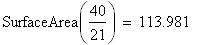Problem
You want to smuggle a precious metal out of the country, by disguising it as a single cylindrical barrel, closed at both ends. The cost of shipping is \$7 per cubic foot. Once out the the country, you can sell the metal for \$8 per square foot. Assuming that you design the barrels with the height equal to twice the diameter, how many square feet should you smuggle, and what will your profit be?
Solution
For a cylinder of radius r and height h,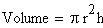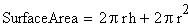Since the height h is to be twice the diameter, and the diameter is twice the radius r,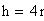and therefore we can write formulas for both the volume and the area in terms of a single variable, r.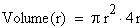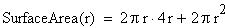The profit will be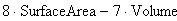As a function of the radius, the profit is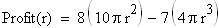To maximize the profit, take its derivative, set it equal to zero, and solve for r.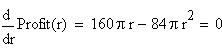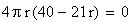This has solutions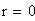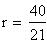The value r = 0 gives a barrel of zero volume and surface area, and a corresponding profit of zero, so it is unlikely to be the correct answer. With r = 40/21, we get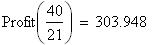The number of square feet of metal needed to produce this profit is